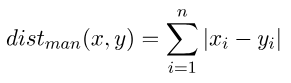# 相似度计算之曼哈顿距离```import numpy as np
def manhattan_distance(p_vec, q_vec):
"""
This method implements the manhattan distance metric
:param p_vec: vector one
:param q_vec: vector two
:return: the manhattan distance between vector one and two
"""
return np.sum(np.fabs(p_vec - q_vec))```